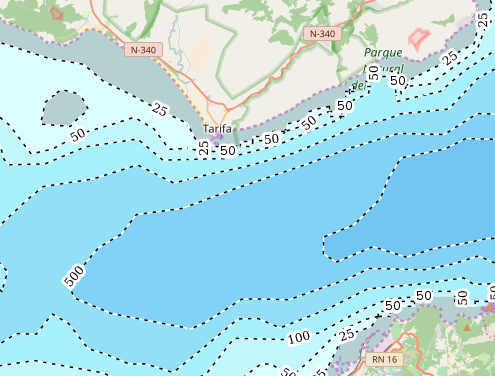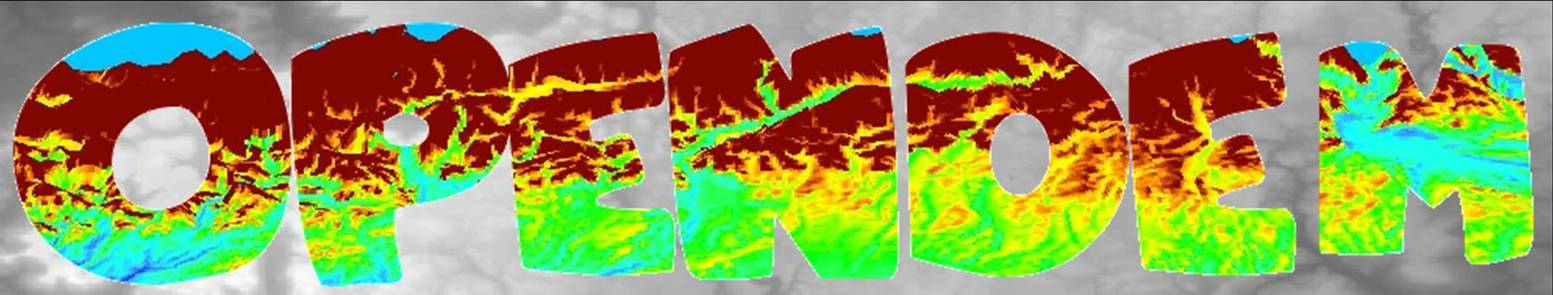The data was derived for OpenSeaMap from the GEBCO_2014 Grid, version 20141103 www.gebco.net, and has use limitations:

"Use of the printed map and/or digital imagery for scientific research, environmental conservation, education or other non-commercial purposes is authorised without the prior permission of the data set originators, providing the source material is properly credited."

Please credit the source properly.

The resolution of the GEBCO 2014 dataset is 30-arc seconds.Fig. 1: Strait of Gibraltar with the downloadable bathymetry data. OSM tiles as background.

A bicubic interpolation of the gebco data was done by the factor 3.

The data was clipped with the OSM landtiles to avoid overlapping.

The data was classified on base of the depth values:

-1 thru -24.99 = 1
-25 thru -49.99 = 2
-50 thru -99.99 = 3
-100 thru -249.99 = 4
-250 thru -499.99 = 5
-500 thru -749.99 = 6
-750 thru -999.99 = 7
-1000 thru -1249.99 = 8
-1250 thru -1499.99 = 9
-1500 thru -1749.99 = 10
-1750 thru -1999.99 = 11
-2000 thru -2499.99 = 12
-2500 thru -2999.99 = 13
-3000 thru -3499.99 = 14
-3500 thru -3999.99 = 15
-4000 thru -4499.99 = 16
-4500 thru -4999.99 = 17
-5000 thru -5499.99 = 18
-5500 thru -5999.99 = 19
-6000 thru -6499.99 = 20
-6500 thru -6999.99 = 21
-7000 thru -7499.99 = 22
-7500 thru -7999.99 = 23
-8000 thru -8499.99 = 24
-8500 thru -8999.99 = 25
-9000 thru -9499.99 = 26
-9500 thru -12000 = 27

Polygons were computed and splitted for a better performance.

Contours were derived.